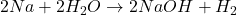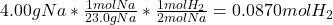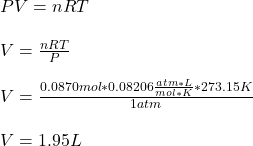## How many liters of hydrogen are obtained from the reaction of 4.00 g sodium with excess water, at STP?

Question

How many liters of hydrogen are obtained from the reaction of 4.00 g sodium with excess water, at STP?

in progress 0
5 months 2021-08-15T13:18:27+00:00 1 Answers 2 views 0

## Answers ( )

V = 1.95 L.

Explanation:

Hell there!

In this case, according to the following reaction between sodium metal and water:We can realize that the moles of hydrogen can be calculated by using the initial mass of sodium, its atomic mass (23.0 g/mol) and the 2:1 mole ratio of sodium to hydrogen to obtain:Finally, we calculate the volume of hydrogen by using the ideal gas equation whereas the pressure is 1 atm and the temperature 273.15 K according to the STP conditions:Regards!